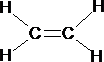# Intermediate Organic Chemistry

Consider carbons electronic configuration once again.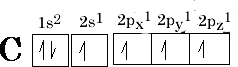We have 4 unpaired electrons in this carbon atom, it is obvious then that the atom will give 4 bonds with other atoms. Consider CH4 , C - H distance is 0.111 nanometres for all of the bonds, surely this cannot be the case as we have 2 different types of bond?! The p orbitals are weaker than s orbital overlap so the bond should be longer! What is happening?

This is a case of hybridisation this makes four equivalent bonds called sp3 molecular orbitals Figure 8(a); this indicates that 1 s and 3 (2p) orbitals have mixed: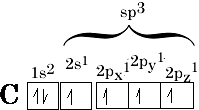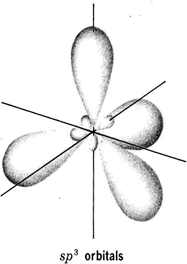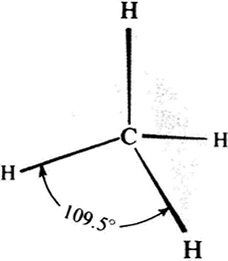Hybridisation means mixing together of the orbitals to form equivalent bonds, the number of orbitals formed must be equal to the number mixing.

If we apply this to CH4 methane we get the result shown in Figure 8(a). Notice the Hydrogen atoms have moved to the farthest point away from each other; this gives a bond angle of 109.50 {Figure 8b}. This is known as the tetrahedral angle.

This is not the only way that hybridisation can occur with the s and p orbitals, i.e. 1(s) and 2 x 2(p) orbitals can mix to form 3sp2 molecular orbitals.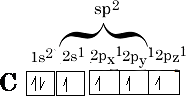The sp2 hybridised orbitals have a similar shape to the sp3 orbitals but we only have 3 orbitals to arrange as far away as possible from one another. The angle they choose is 1200 , what we get this time is a trigonal shape {figure 10};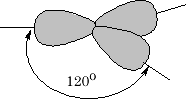This only leaves the 2pz orbital to be arranged as follows: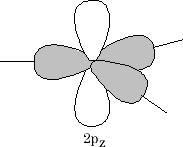The 2p z orbital is now orthogonal to the 3 sp 2 orbitals {figure11}. What we have now is the set-up for the formation of a double bond in an alkene. Two sp 2 orbitals and 2 p orbitals for the carbon atoms need to come together; the resultant is the formation of a s bond and a p bond (figure 12).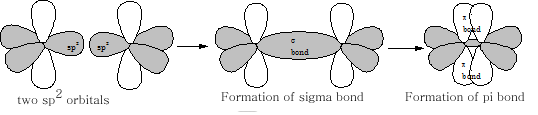The resultant molecule which just requires the overlap of four Hydrogen 1s orbitals with each of the remaining 4 sp2 orbitals and we have the first of the alkenes  ethene: